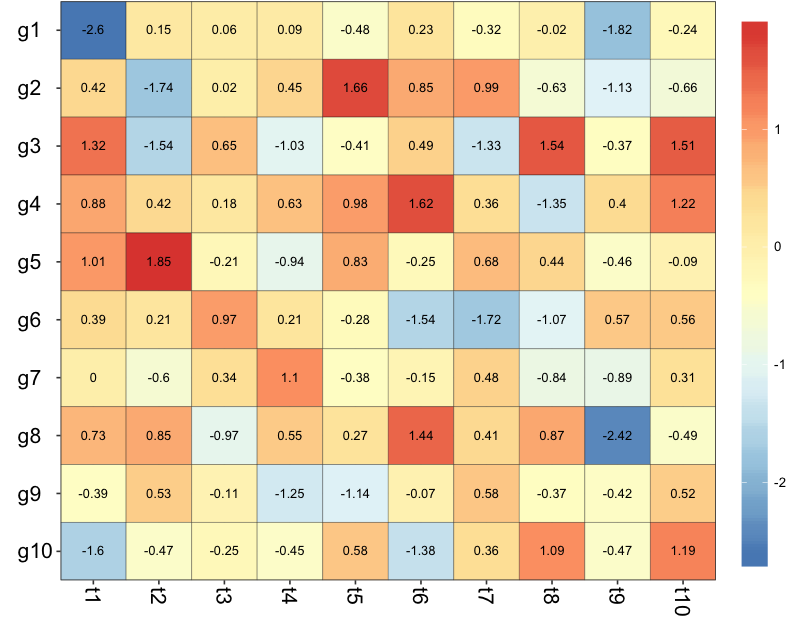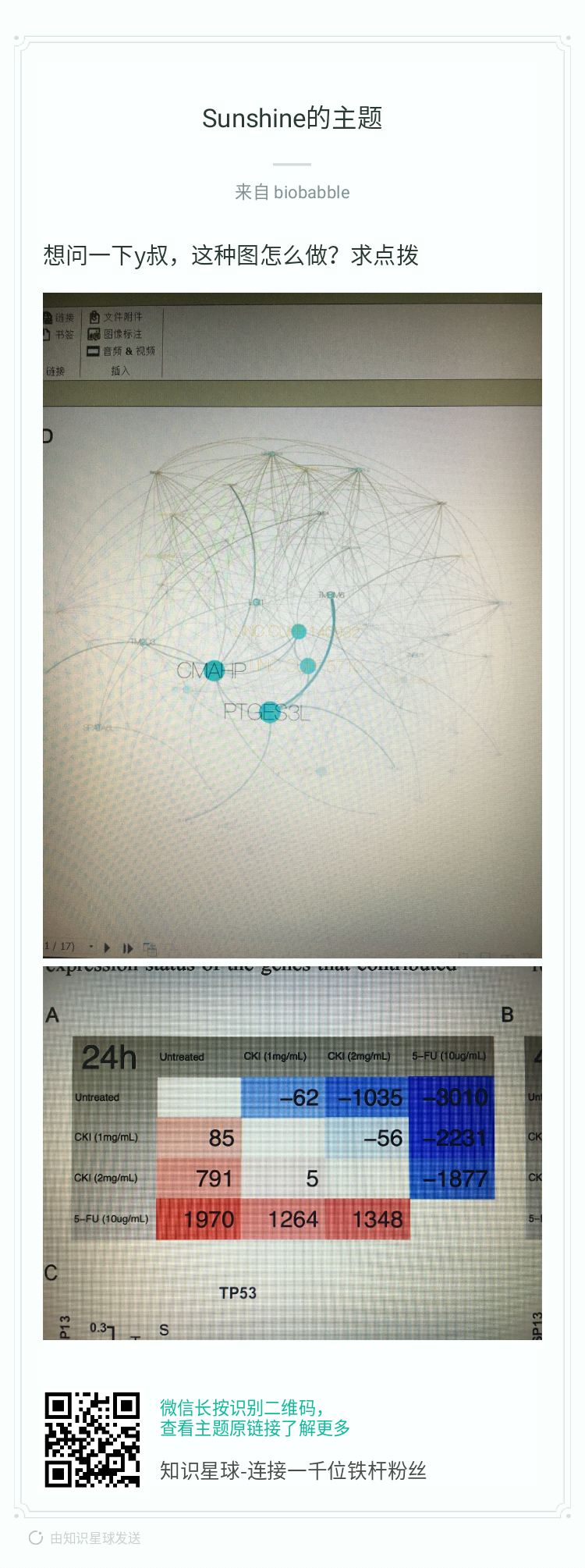Let’s not kid ourselves: the most widely used piece of software for statistics is Excel. – Brian D. Ripley (‘Statistical Methods Need Software: A View of Statistical Computing’) Opening lecture RSS 2002, Plymouth (September 2002)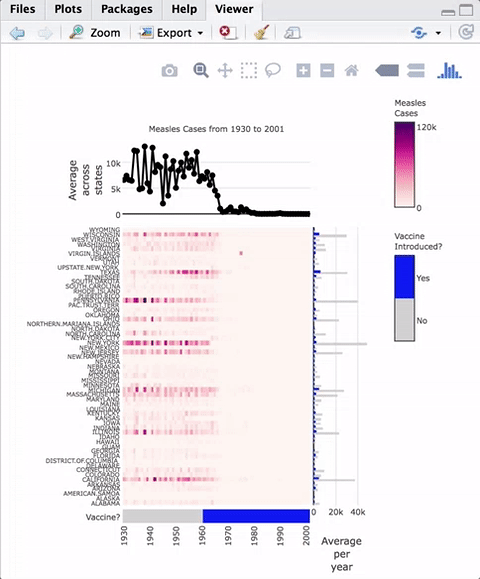``````d = data.frame(matrix(rnorm(100), ncol=10))
colnames(d) = paste0('t', 1:10)
rownames(d) = paste0('g', 1:10)
library(pheatmap)
pheatmap(d) -> x
``````

``````p  = ggplot(d, aes(t1, t2)) + geom_point(size=5)
plot_grid(x\$gtable, p, labels=c('A', 'B'))
``````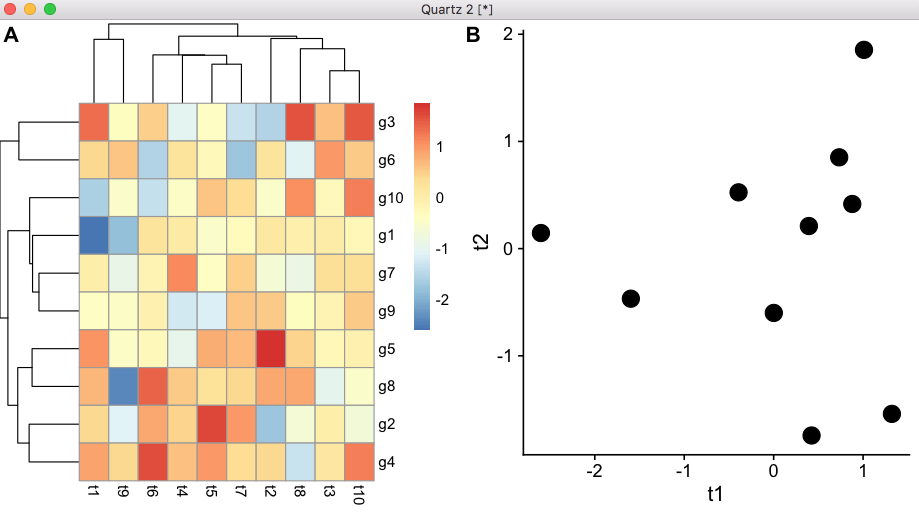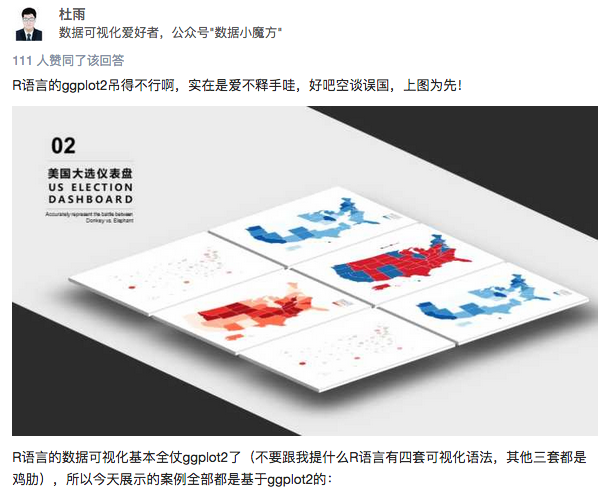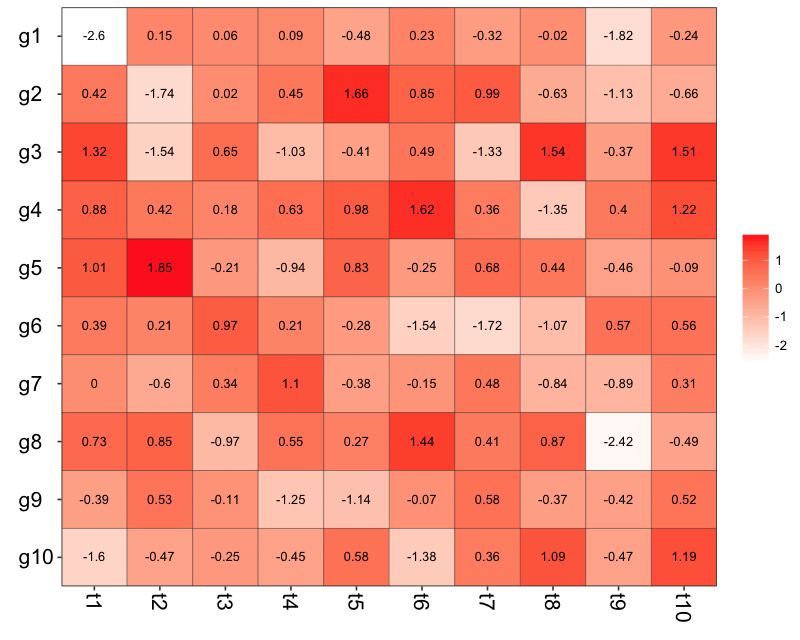``````library(RColorBrewer)
colorRampPalette(rev(brewer.pal(n = 7,
name = "RdYlBu"))) -> cc
``````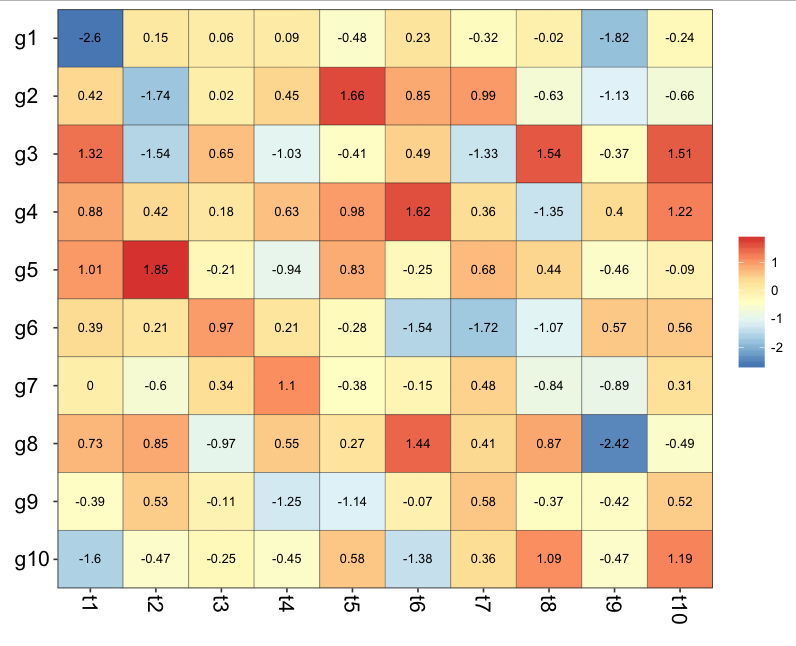``````simplot(d) +